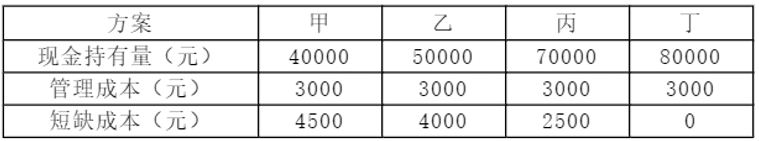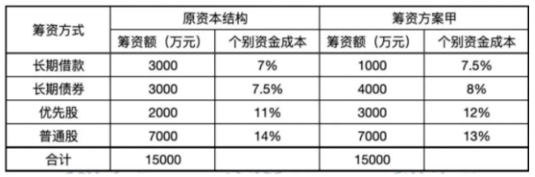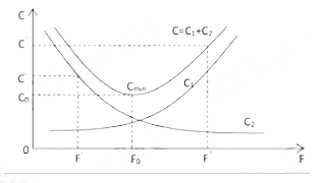120:002022年《建设工程经济》真题（精编）

1
(单项选择题)

• A.

3003.86

• B.

3000.00

• C.

3018.03

• D.

3185.03

• A
• B
• C
• D

2
(单项选择题)

• A.

经济效果评价应定性分析和定量分析相结合，以定性分析为主

• B.

经济效果动态分析不能全面地反映技术方案整个计算期的经济效果

• C.

融资前经济效果分析通常以，动态分析为辅

• D.

方案实施前经济效果分析通常存在—定的不确定性和风险性

• A
• B
• C
• D

3
(单项选择题)

• A.

资金的时间价值是资金随时间周转使用的结果

• B.

资金的时间价值与资金数量无关

• C.

资金的时间价值与资金周转的速度成反比

• D.

利率是衡量资金时间价值的绝对尺度

• A
• B
• C
• D

4
(单项选择题)

• A.

境外投资项目基准收益率的测定，可忽略国家风险因素

• B.

财务基准收益率必须由政府投资主管部门统一确定

• C.

财务基准收益率的确定应考虑资金成本、投资机会成本、通货膨胀和风险因素

• D.

财务基准收益率是投资项目可能获得的最高盈利水平

• A
• B
• C
• D

5
(单项选择题)

• A.

偿债备付率大于1，说明偿付债务本息的能力不足

• B.

偿债备付率从付息资金来源的角度反映企业偿付债务利息的能力

• C.

偿债备付率是还本付息的资金与当期应还本金额的比值

• D.

偿债备付率应在借款偿还期内分年计算

• A
• B
• C
• D

6
(单项选择题)

• A.

现值一定，计息期数相同，折现率越高，终值越小

• B.

现值一定，折现率相同，计息期数越少，终值越大

• C.

终值一定，折现率相同，计息期数越多，现值越大

• D.

终值一定，计息期数相同，折现率越高，现值越小

• A
• B
• C
• D

7
(单项选择题)

• A.

应分利润

• B.

销项税额

• C.

建设投资

• D.

借款利息支付

• A
• B
• C
• D

8
(单项选择题)

• A.

债券利息

• B.

代理发行费

• C.

印刷费

• D.

公证费

• A
• B
• C
• D

9
(单项选择题)

• A.

固定总价合同

• B.

固定单价合同

• C.

可调单价合同

• D.

成本加酬金合同

• A
• B
• C
• D

10
(单项选择题)

• A.

资本积累率

• B.

净资产收益率

• C.

销售净利率

• D.

总资产周转率

• A
• B
• C
• D

11
(单项选择题)

• A.

6

• B.

1

• C.

5

• D.

4

• A
• B
• C
• D

12
(单项选择题)

• A.

④→②→③→⑥→①→⑤

• B.

④→②→③→①→⑤→⑥

• C.

④→①→③→②→⑥→⑤

• D.

④→①→②→③→⑥→⑤

• A
• B
• C
• D

13
(单项选择题)

• A.

1156.2

• B.

600.0

• C.

886.2

• D.

1772.4

• A
• B
• C
• D

14
(单项选择题)

• A.

其他项目清单中应列出总承包服务费

• B.

暂估价应列出材料暂估价和工程设备暂估价，不考虑专业工程暂估价

• C.

暂列金额一般应尽可能列高，以避免在实际中超出该数量

• D.

计日工应按照招标工程的复杂程度估算一个数量，该数量一般要比实际

• A
• B
• C
• D

15
(单项选择题)

• A.

结构复杂、性能和技术指标较差的工程产品

• B.

用户意见少且竞争力较强的工程产品

• C.

成本较低或占总成本比重较小的工程产品

• D.

工艺简单、原材料能耗较低、质量有一定保障的工程产品

• A
• B
• C
• D

16
(单项选择题)

• A.

投标人按规范修正后的项目特征

• B.

投标人参照以往完成的实际施工的项目特征

• C.

招标文件中的施工图纸

• D.

招标工程量清单的项目特征描述

• A
• B
• C
• D

17
(单项选择题)

• A.

211

• B.

222

• C.

2110

• D.

2220

• A
• B
• C
• D

18
(单项选择题)

• A.

监理人应在收到承包人提交的工程量报告后的14天内完成对承包人提交的工程量报告的审核并报送发包人

• B.

监理人未在收到承包人提交的工程量报表后的5天内完成复核的，承包人提交的工程量报告中的工程量视为承包人实际完成的工程量

• C.

承包人未按监理人要求参加工程量复核或抽样复测的，监理人复核或修正的工程量视为承包人实际完成的工程量

• D.

承包人为保证施工质量超出施工图纸范围施工的工程量，监理人应予以计量

• A
• B
• C
• D

19
(单项选择题)

• A.

参照类似项目的单价认定

• B.

按照直接成本加适当利润的原则，由发包人确定变更单价

• C.

按照合理的成本加利润的原则，由承包人确定变更工作的单价

• D.

根据合理的成本加适当利润的原则，由监理人确定新的变更单价

• A
• B
• C
• D

20
(单项选择题)

• A.

人工费

• B.

措施项目费

• C.

企业管理费

• D.

规费

• A
• B
• C
• D

21
(单项选择题)

• A.

企业所在地社会平均工资

• B.

企业所在地预算人工价格

• C.

地区劳务市场价格

• D.

企业所在地最低工资标准

• A
• B
• C
• D

22
(单项选择题)

• A.

存货破损和变质损失

• B.

材料供应中断造成的停工损失

• C.

丧失销售机会的损失

• D.

产成品库存缺货造成的拖欠发货损失

• A
• B
• C
• D

23
(单项选择题)

• A.

计入投标总价，由承包人决定使用

• B.

不计入投标总价，实际发生时业主支付

• C.

计入投标总价，但承包人无权自主使用

• D.

不计入投标总价，由工程师决定使用

• A
• B
• C
• D

24
(单项选择题)• A.

• B.

• C.

• D.

• A
• B
• C
• D

25
(单项选择题)

• A.

19

• B.

17

• C.

20

• D.

27

• A
• B
• C
• D

26
(单项选择题)

• A.

混凝土模板费

• B.

安全文明施工费

• C.

材料二次搬运费

• D.

已完工程及设备保护费

• A
• B
• C
• D

27
(单项选择题)

• A.

有利于保证和提高产品质量，提高自动化程度

• B.

对产品的质量性能和方案的生产能力有足够的保证程度

• C.

必须考虑是否会对操作人员造成人身伤害，有无保护措施

• D.

充分发挥企业和方案所在地的资源优势

• A
• B
• C
• D

28
(单项选择题)

• A.

资产和负债

• B.

资产和所有者权益

• C.

货币资金和所有者权益

• D.

负债和所有者权益

• A
• B
• C
• D

29
(单项选择题)

• A.

4410

• B.

4200

• C.

4720

• D.

5040

• A
• B
• C
• D

30
(单项选择题)

• A.

18.5

• B.

19.5

• C.

20

• D.

16.5

• A
• B
• C
• D

31
(单项选择题)

• A.

谨慎

• B.

一贯性

• C.

及时性

• D.

配比

• A
• B
• C
• D

32
(单项选择题)

• A.

提供专利使用所得的收入

• B.

转让财产收入

• C.

接受捐赠取得的收入

• D.

接受财政拨款取得的收入

• A
• B
• C
• D

33
(单项选择题)

• A.

1.67

• B.

1.05

• C.

1.24

• D.

2.14

• A
• B
• C
• D

34
(单项选择题)

• A.

安全文明施工费和企业管理费

• B.

规费和企业管理费

• C.

措施项目费和规费

• D.

规费和税金

• A
• B
• C
• D

35
(单项选择题)

• A.

工程概况

• B.

工程招标及分包范围

• C.

工程单清单编制依据．

• D.

工程质量材料施工等的特殊要求

• A
• B
• C
• D

36
(单项选择题)

• A.

按照实际使用材料价格换算定额单价

• B.

编织补充定价单额

• C.

直接套用定额单价

• D.

调量不换价

• A
• B
• C
• D

37
(单项选择题)

• A.

不确定因素的临界点越低，该因素对技术方案的评价指标影响越小

• B.

敏感性分析可以通过计算敏感度系数和临界点确定敏感因素

• C.

敏感度系数大于零，表示评价指标与不确定因素反方向变化

• D.

敏感度系数的绝对值越大，表明评价指标对于不确定因素越不敏感

• A
• B
• C
• D

38
(单项选择题)• A.

• B.

• C.

• D.

• A
• B
• C
• D

39
(单项选择题)

• A.

价值变动风险小

• B.

投资数额小

• C.

预期收益高

• D.

转换方式多

• A
• B
• C
• D

40
(单项选择题)

• A.

将原合同未履约部分与合同变更部分合并为新合同进行会计处理

• B.

将该合同变更部分作为原合同的组成部分进行会计处理

• C.

将该合同变更部分作为一份单独的合同进行会计处理

• D.

将原合同已履约部分与合同变更部分合并为新合同进行会计处理

• A
• B
• C
• D

41
(单项选择题)• A.

随着产品功能水平F提高，生产成本C1降低，使用及维护成本C2增加

• B.

在F处，产品的功能较少，生产成本C1、使用及维护成本C2较低，寿命周期成本较低

• C.

在F’处，产品功能较多，生产成本C1、使用及维护成本C2均较高，寿命周期成本较高

• D.

在F0时，产品满足必要的功能需求，生产成本C1、使用及维护成本C2之和对应的寿命周期成本最低

• A
• B
• C
• D

42
(单项选择题)

• A.

暂列金额、规费、税金

• B.

安全文明施工费、规费、税金

• C.

暂估价、规费、税金

• D.

计日工、安全文明施工、税金

• A
• B
• C
• D

43
(单项选择题)

• A.

32

• B.

35

• C.

37

• D.

41

• A
• B
• C
• D

44
(单项选择题)

• A.

计算人工费、材料费和施工机械使用费的方法不同

• B.

计算工程量的方法不同

• C.

计算企业管理费的方法不同

• D.

计算其他税费的程序不同

• A
• B
• C
• D

45
(单项选择题)

• A.

45%

• B.

48%

• C.

55%

• D.

40%

• A
• B
• C
• D

46
(单项选择题)

• A.

奖金

• B.

特殊情况下支付的工资

• C.

津贴补贴

• D.

加班加点工资

• A
• B
• C
• D

47
(单项选择题)

• A.

31.11%

• B.

63.49％

• C.

31.42%

• D.

62.22%

• A
• B
• C
• D

48
(单项选择题)

• A.

投标人均应按工程量清单列出的项目不加修改地投标

• B.

工程量清单列出的工程项目内容、数量和质量要求是投标人竞争的共同基础

• C.

投标人均按工程量清单中确定的计量规划计算工程量

• D.

工程量清单中的项目和综合单价是投标人平等竞争的基础和依据

• A
• B
• C
• D

49
(单项选择题)

• A.

14000

• B.

15000

• C.

16000

• D.

10000

• A
• B
• C
• D

50
(单项选择题)

• A.

18

• B.

5

• C.

8

• D.

20

• A
• B
• C
• D

51
(单项选择题)

• A.

467.72

• B.

537.44

• C.

502.58

• D.

542.86

• A
• B
• C
• D

52
(单项选择题)

• A.

预付款担保不宜采用银行保函和担保公司相保形式

• B.

发包人在中期扣回预付款后，剩余的预付款担保金额可低于未被扣回的预付款金额

• C.

在颁发工程接收证书前，提前解除合同的，尚未扣回的预付款应与合同价款一并结算

• D.

发包人逾期支付预付款超过7天的，承包人有权暂停施工

• A
• B
• C
• D

53
(单项选择题)

• A.

通过监理工程师下达变更指令要求承包人必须提前峻工，并支付由此增加的提前竣工费用

• B.

增加合同补充条款要求承包人采取加快工程进度措施，发包人不承担赶工费用

• C.

征得承包人同意后，与承包人商定采取加快工程进度的措施，并承担承包人由此增加的提前竣工费用

• D.

发承包双方应签订补充合同约定提前竣工的赶工费用总额，并各承担50%的费用

• A
• B
• C
• D

54
(单项选择题)

• A.

按照投标人自行修正的工程量填报单价，另在投标函中予以说明

• B.

电话咨询招标人，根据招标人口头认可的工程量填报单价

• C.

按照原招标文件的工程量填报单价，可另在投标函中予以说明

• D.

按照施工中可能发生的工程量填报单价，另在投标函中予以说明

• A
• B
• C
• D

55
(单项选择题)

• A.

工人偶然违背劳动纪律造成的损失时间

• B.

材料供应不及时造成的停工时间

• C.

劳动组织不合理引起的停工时间

• D.

工人手工操作的辅助工作时间

• A
• B
• C
• D

56
(单项选择题)

• A.

分部分项工程量清单

• B.

其他项目清单

• C.

工程量清单编制总说明

• D.

工程量清单编制依据

• A
• B
• C
• D

57
(单项选择题)

• A.

单位工程中各分部分项工程的保修期必须相同

• B.

保修期内，承包人应承担全部工程损坏的维修责任

• C.

工程保修期从工程竣工验收合格之日起算

• D.

发包人未经竣工验收擅自使用工程的，保修期自使用之日起算

• A
• B
• C
• D

58
(单项选择题)

• A.

需适应投标企业的资质等级、规模和采取的特殊施工方案

• B.

需考虑拟建工程施工现场可能出现的零星工作

• C.

可参考拟建工程的常规施工组织设计和施工技术方案

• D.

不考虑设计文件中不足以写进施工方案但要通过技术措施才能实现的内容

• A
• B
• C
• D

59
(单项选择题)

• A.

6234.255

• B.

4725.000

• C.

5625.000

• D.

5719.500

• A
• B
• C
• D